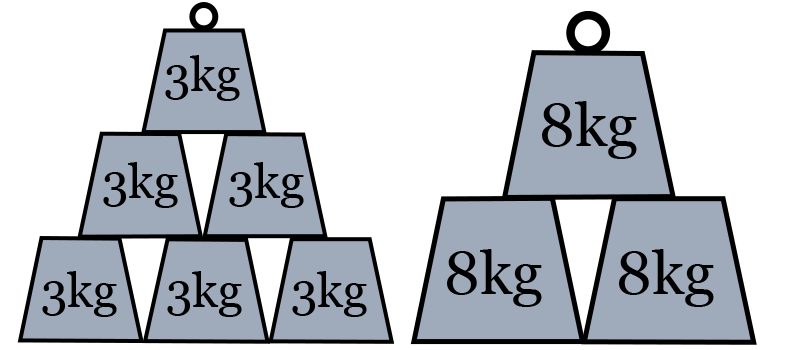# Weighty AveragesLet's suppose that you have a large supply of both $3$kg weights and $8$kg weights.By combining weights, you could make sets with different average (mean) weights...

Two $3$kg weights and three $8$kg weights have a mean weight of $6$kg.

Can you find other combinations of $3$kg and $8$kg weights whose mean weight is a whole number of kg?

What's the smallest?

What's the largest?

Can you make all the whole numbers in between?

Instead of $3$kg and $8$kg, you could choose two different weights - $2$kg and $7$kg, for example.

Choose some different pairs of weights. Which whole numbers is it possible to have as the mean weight?

Can you devise an efficient strategy to work out the number of weights of each type needed to get any mean weight?

What about finding mean weights that are not a whole number of kg?